# 论文引入

• 提出了一种Hierarchical GAN架构，用于从面部照片中进行艺术人像合成，可以生成高质量和富有表现力的艺术肖像画。特别是，可以用细腻的白线学习复杂的发型。

• 为了最好地模拟艺术家，模型将GAN的渲染输出分成多个层，每个层由分离的损失函数控制。
• 从10个面部数据集中收集的6,655张正面照片预训练模型，并构建适合训练和测试的APDrawing数据集（包含140张专业艺术家的高分辨率面部照片和相应的肖像画）。

# 模型结构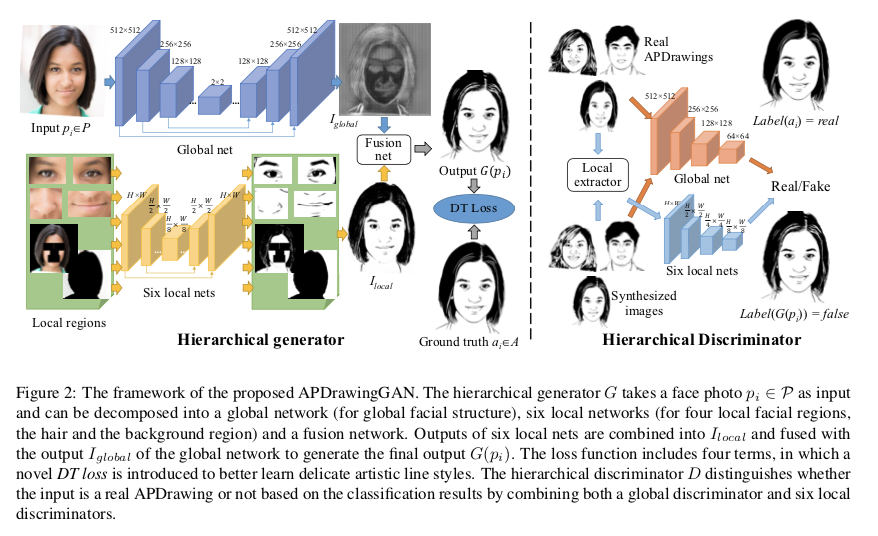## 损失函数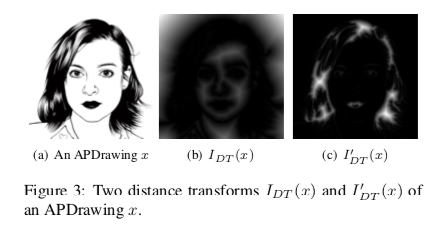$d_{CM}(a_i,G(p_i))=\sum_{(j,k) \in \Theta_b(a_i)}I_{DT}(G(p_i))(j,k) + \sum_{(j,k) \in \Theta_\omega(a_i)}I^{'}_{DT}(G(p_i))(j,k)$

$L_{DT}(G,D) = \mathbb E_{(p_i,a_i) \sim S_{data}}[d_{CM}(a_i,G(p_i))+d_{CM}(G(p_i),a_i)]$

$\min_G \max_D L(G,D) = L_{adv}(G,D)+\lambda_1 L_{\mathcal L_1}(G,D)+\lambda_2 L_{DT}(G,D)+\lambda_3 L_{local}(G,D)$

# 实验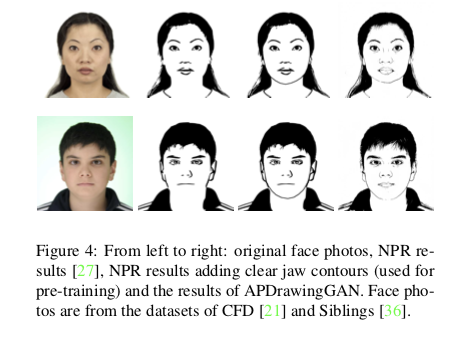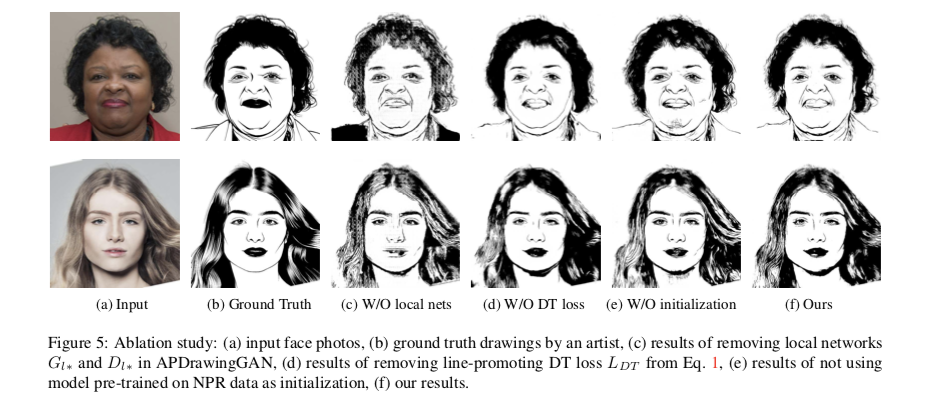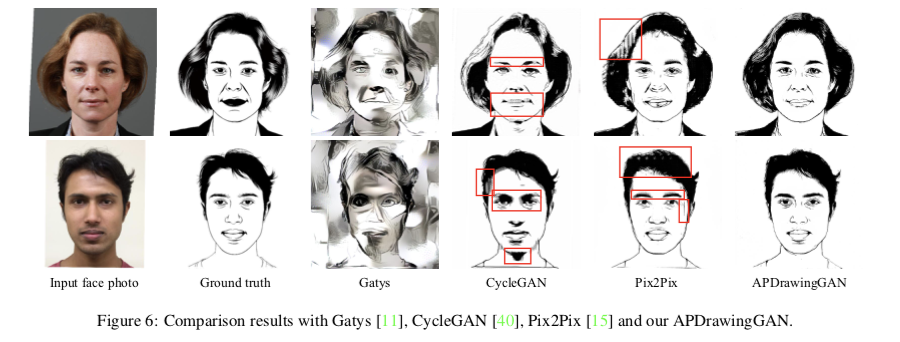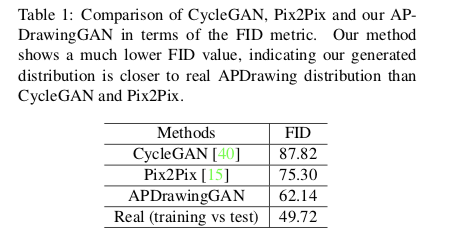# 参考文献

 Paul L. Rosin and Yu-Kun Lai. Towards artistic minimal rendering. In International Symposium on Non-Photorealistic Animation and Rendering, NPAR ’10, pages 119–127, 2010. 5, 6

 Brandon Amos, Bartosz Ludwiczuk, and Mahadev Satyanarayanan. OpenFace: A general-purpose face recognition library with mobile applications. Technical report, CMUCS-16-118, CMU School of Computer Science, 2016. 6